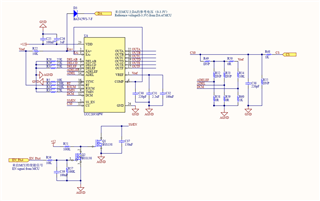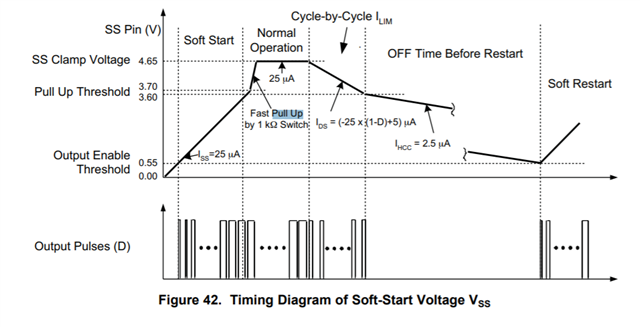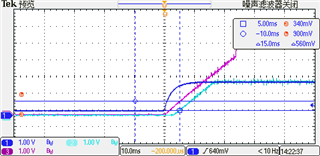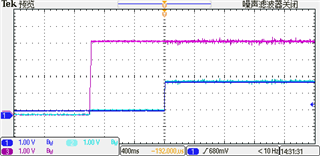# UCC28950: Several questions about starting UCC28950

Part Number: UCC28950

hi TI，

I am making a 55A58V charger, which requires 0-100% output voltage and current, so I use MCU and UCC28950 to build the control framework. The MCU generates the softstart/enable siganl to SS/EN pin and reference voltage to EA+ pin  for UCC28950. The UCC28950 generates the PWM pulses for PSFB. The operational amplifier inside UCC28950 is designed as a voltage follower. I will adjust the voltage on EA+ to affect the phase shift angle to adjust the output voltage.

The detailed design of UCC28950 is shown as following pic.Q1: If the MCU provides SS/EN and reference voltage at the same time, the oscilloscope shows that the voltage of EA+ (cyan curve) quickly rises to the set voltage, while the voltage on SS/EN(blue curve) gradually rises to the maximum voltage. I think that due to the SS/EN voltage, the phase shift angle should gradually increase from zero to the angle set by EA+. But in reality, the phase shift angle reaches its maximum value, which means that the output reaches its maximum value and then decreases to the angle set by EA+.wHY?Q2: If I give the start signal first, delay for a period of time (such as 100ms), and then give the reference voltage of EA+, there will be no situation encountered in question 1. The phase shift angle will jump directly from zero to the angle set by EA+. WHY?

Q3:From the above waveforms, it can be seen that the voltage on the SS/EN pin rises linearly from 0V at first, but it will suddenly rise to full scale later. Why?

• Hi, Cheng

1. regarding to Q1 and Q2, could you capture VCOMP with SS/EN and EA+ together, sine  the duty cycle is defined by VCOMP only.

2. regarding to Q3, SS/EN is pull up to 4.7V if SS/EN above 3.7V. So your observation is right.Yunsheng

• Hi, yunsheng

Thanks for your reply.

I'm clear about my Q3.

I've not measured the voltage on COMP yet because I use the op amp inside of UCC28950 as a voltage follower which means the voltage on COMP should be same as EA+. I'll try to capture it following your advise.

Thank you so much.

• Hi, Cheng

looking forward to see the waveform.

Yunsheng

• Hi, yunsheng

Here are some waveforms on COMP, EA+ and SS/EN. The blue curve is EA+, the cyan curve is COMP and the purple curve is SS/EN

1. This waveform appears when MCU gives SS/EN and EA+ at the same time.2. This waveform appears when MCU gives SS/EN at first and  EA+ after 1S.From the above pictures, the voltage on COMP is always the same as the smaller one between EA+ and SS/EN(SS/EN-0.55V).

Is there anything abnormal?